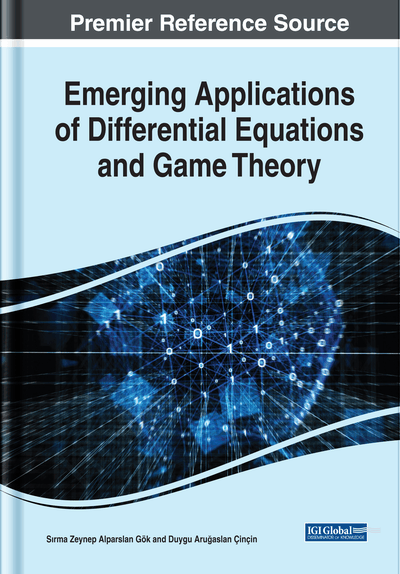# Application of the Exponential Rational Function Method to Some Fractional Soliton Equations

Mustafa Ekici (Usak University, Turkey) and Metin Ünal (Usak University, Turkey)
DOI: 10.4018/978-1-7998-0134-4.ch002
Available
\$37.50
No Current Special Offers

## Abstract

In this chapter, the authors study the exponential rational function method to find new exact solutions for the time-fractional fifth-order Sawada-Kotera equation, the space-time fractional Whitham-Broer-Kaup equations, and the space-time fractional generalized Hirota-Satsuma coupled KdV equations. These fractional differential equations are converted into ordinary differential equations by using the fractional complex transform. The fractional derivatives are defined in the sense of Jumarie's modified Riemann-Liouville. The proposed method is direct and effective for solving different kind of nonlinear fractional equations in mathematical physics.
Chapter Preview
Top

## Introduction

Fractional calculus deals with the derivatives and integrals of any real or complex order (Greenberg, 1978; Podlubny, 1999; Hilfer, 2000). This type of calculus was initiated and developed by many great scientists like Leibniz, L’Hospital, Euler, Bernoulli, Riemann, Liouville, and many others (Kilbas, Srivastava, & Trujillo, 2006). Many researchers have been working to develop the fractional calculus and make use of its applicability to various areas of mathematics, physics and engineering. It has been found that fractional calculus can be extensively used for many physical phenomena as a strong and effective tool to describe mathematical modeling (Caputo, & Fabrizio, 2015; Erbe, Goodrich, Jia, & Peterson, 2016).

In recent years, fractional differential equations (FDEs) have been popular among the researchers and gained much attention. FDEs are generalization of classical differential equations of integer order. They are widely used to describe various complex phenomena such as in fluid flow, control theory, signal processing, systems identification, viscoelasticity, acoustic waves etc. The exact solutions of nonlinear fractional differential equations by using various different methods have been investigated by many researchers. Many useful method for finding exact solutions of FDEs have been proposed. Such as the tanh-function expansion method (Fan, 2000), the Jacobi elliptic function expansion (Liu, Fu, Liu, & Zhao, 2001), the homogeneous balance method (Wang, 1995; Wang, 1996; Wang, Zhou, &Li, 1996), the trial function method (Kudryashov, 1990), the exponential function method (He & Wu, 2006), the ()-expansion method (Zhang, Tong, & Wang, 2008), the sub-ODE method (Zhang, Wang, & Li, 2006; Wang, Li, & Zhang, 2007), Adomian decomposition method (El-Sayed & Gaber, 2006; El-Sayed, Behiry, & Raslan, 2010), the homotopy analysis method (Arafa, Rida, & Mohamed, 2011), the differential transformation method (Odibat & Momani, 2008), the fractional sub-equation method (S. Zhang & H. Q. Zhang, 2011; Guo, Mei, Li, & Sun, 2012; Lu, 2012) and so on.

There are several types of fractional derivatives, among the popular of them are given in the following sections.

### Riemann-Liouville Fractional Derivatives

The Riemann-Liouville fractional integration operator is defined as

where a>0, t>α, a,t,α∈R (Gorenflo & Mainardi, 2000). The Riemann-Liouville fractional differential operator is defined aswhere a>0, t>α, a,t,α∈R (Gorenflo & Mainardi, 2000). The well-known fractional integral is the Riemann-Liouville type which is based on the generalisation of the usual Riemann integral(Jarad, Abdeljawad, & Baleanu, 2012).

## Complete Chapter List

Search this Book:
Reset